# Do It Yourself LSTM with TensorFlow

After reading the great post Understanding LStM Networks by Christopher Olah, I was curious how an actual implementation would look like in TensorFlow, without using its built-in `nn.rnn_cell.LSTMCell`.

Below you'll find an implementation explained with Christopher Olah's words and illustrations. If you haven't already you might want to go to the original and read the whole post to understand what LSTMs are and how they are working in general before diving into details here.

Keep in mind that this is probaly nothing you'll want to use in some real world scenario, since you'll achieve better results with TensorFlow's own LSTM and RNN implementations (if you're interested in that, checkout another notebook I've made). This post is intended for educational purposes only, if you want, like me, to learn how a LSTM is working

You can download this post as a Jupyter playbook from the GitHub repo.

## 1. Example Usecase

Let's say I'm showing you different combinations of the signs `+`, `-`, `0` and `I`, end tell you for every sequence the resulting sum:

• `+++``3`
• `--``-2`
• `+--``-1`
• `00+``1`
• `I+``-1`
• `-``-1`
• `I0-``1`
• `-I--``-1`
• ...

You'd probaly get pretty quickly that `+` means "plus 1", `-` means "minus 1", `0` means "do nothing" and `I` inverts the meaning of following characters.

As soon as you identify these meanings I could give you arbitrarily long sequences, one sign at a time, and you were able to tell me the current sum at each step. But playing this with humans gets boring for everyone involved pretty quickly and would miss the point of this post, so let's rather take this as an example use case for our LSTM endeavour: Our goal is to build a LSTM with TensorFlow, get it to understand the meaning of those signs and enable it to calculate the sum at each step in an arbitrarily chosen sequences.

## 2. The Data

I built a simple script that generates all possible combinations of `+`, `-`, `0` and `I`. To keep it simple we'll use a sequence length of 6, which will result in `6^4 = 4096` combinations. Those combinations get shuffled by the data generator, but since I fixed the seed of the randomizer, it will always generate them in the same "random" order.

``````import numpy as np
from pprint import pprint
import datetime

import data_generator

sequence_length = 6

reference_input_data, reference_output_data = data_generator.getSequences(sequence_length)

# data_generator.getSequences(sequence_length) generates all possible combinations of
# the characters '+-0I', so for a sequence length of 6 characters there are a
# a total of 4^6 = 4096 possible combinations. Some Examples:
# '+-+-+-' = 0
# '------' = -6
# '0++000' = 2
# 'I++000' = -2

print('Those sequences are encoded: Every character is representated by a vector, \n\
so the actual return value from data_generator.getSequences looks like this:')
pprint(reference_input_data)

print('There is a helper to decode that again:')
pprint(data_generator.decodeSequence(reference_input_data))

print('The solution for that sequence is:')
print(reference_output_data)

instruction_count = np.array(reference_input_data).shape
``````

This gets us:

``````    Those sequences are encoded: Every character is representated by a vector,
so the actual return value from data_generator.getSequences looks like this:
array([[0, 0, 0],
[1, 0, 0],
[0, 0, 1],
[0, 0, 0],
[0, 0, 0],
[0, 0, 0]])
There is a helper to decode that again:
'0+I000'
The solution for that sequence is:
1
``````

Since it would be too easy for our network to learn by looking at all examples and we also want to prevent overfitting, we only take a subset (one fourth) for the actual training. The network should be able to deal with a much smaller training set, but then it needed more training iterations.

``````NUM_EXAMPLES = len(reference_input_data) / 4 # we use 1/4 of the data for the training

test_input = reference_input_data[NUM_EXAMPLES:]
test_output = reference_output_data[NUM_EXAMPLES:] # everything beyond NUM_EXAMPLES

train_input = reference_input_data[:NUM_EXAMPLES]
train_output = reference_output_data[:NUM_EXAMPLES]

print("The network will be trained with " + str(NUM_EXAMPLES) + " out of " + str(len(reference_input_data)) + " Examples")
``````
``````import tensorflow as tf

data = tf.placeholder(tf.float32, [None, sequence_length, instruction_count], name='data')
target = tf.transpose(tf.placeholder(tf.float32, [None], name='target'))
``````

## 3. LSTM Layer

Before we start building the individual layers, we need to set the size of the conveyor, which is typically referred to as the the size "of the LSTM". I'm also setting the feature size here, although this information could also be inferred from the input data.

``````LSTM_SIZE = 24
FEATURE_SIZE = 3 # this is 3 because we use a 3D-vector to represent +, -, 0 and I
``````

Thankfully all weights and biases we need in the LSTM have the same shape - so we can build a small helper function that will save us some code.

The weights get initialized with values between `-0.4` and `0`. Initially I started with values between `-1` and `1`, but these values result in a much better training rate. I'm not exactly sure why that is, but my theory is that this way in the beginning nothing gets "through" the LSTM at all, so the network gradually allows the relevant information to get through – but this guess is definitely a long shot.

``````def default_weights_and_bias():
Weights = tf.Variable(tf.truncated_normal([LSTM_SIZE, LSTM_SIZE + FEATURE_SIZE], -0.2, 0.1))
bias = tf.Variable(tf.constant(0.0, shape = [LSTM_SIZE, 1]))

return Weights, bias
``````

### 3.1 Forget Layer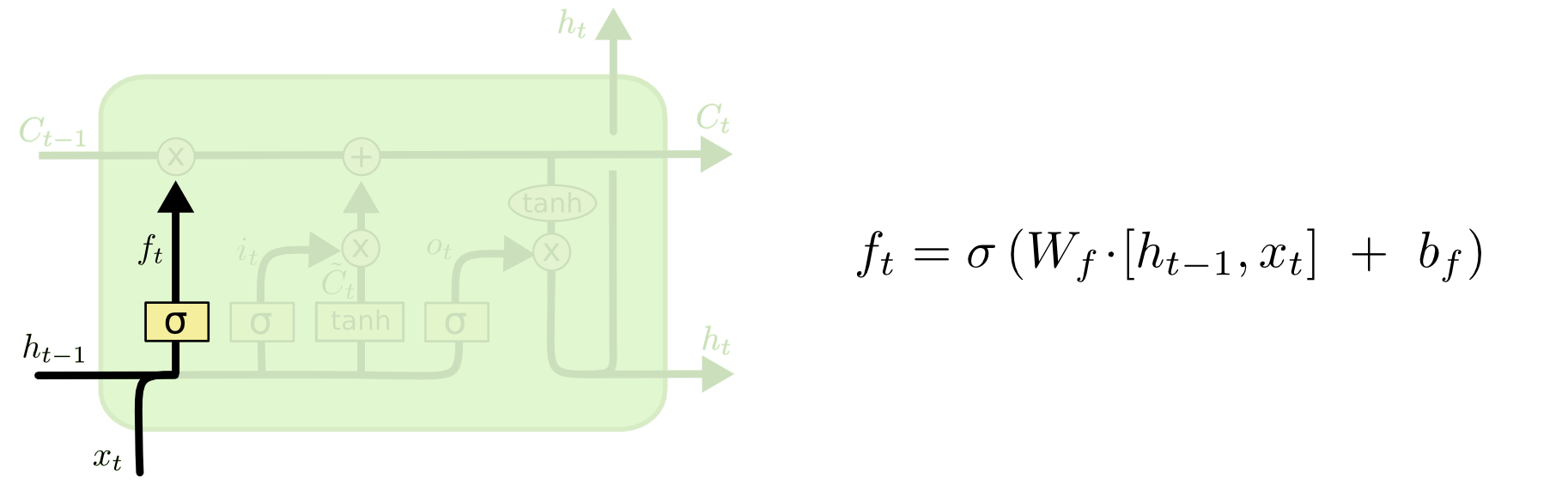The first step in our LSTM is to decide what information we’re going to throw away from the cell state. This decision is made by a sigmoid layer called the “forget gate layer.” It looks at ht−1 and xt, and outputs a number between `0` and `1` for each number in the cell state Ct−1. A 1 represents "completely keep this" while a 0 represents "completely get rid of this."

One important thing here: This is the only layer where we want our bias to be initialized with `1`, so the network will initially keep all states and is thereby forced to deal with values set to the conveyor. This results in a faster learning rate.

``````W_f, _ = default_weights_and_bias()

b_f = tf.Variable(tf.constant(1.0, shape = [LSTM_SIZE, 1]))

# The forget layer
#
# Shapes:
#   - W_f: 24x27
#   - ht_minus_1_and_xt: 27x?
#   - b_f: 24x1
#   - f_t: 24x?
def f_t(ht_minus_1_and_xt):
return tf.sigmoid(tf.matmul(W_f, ht_minus_1_and_xt) + b_f)
``````

### 3.2 New Candidate Conveyor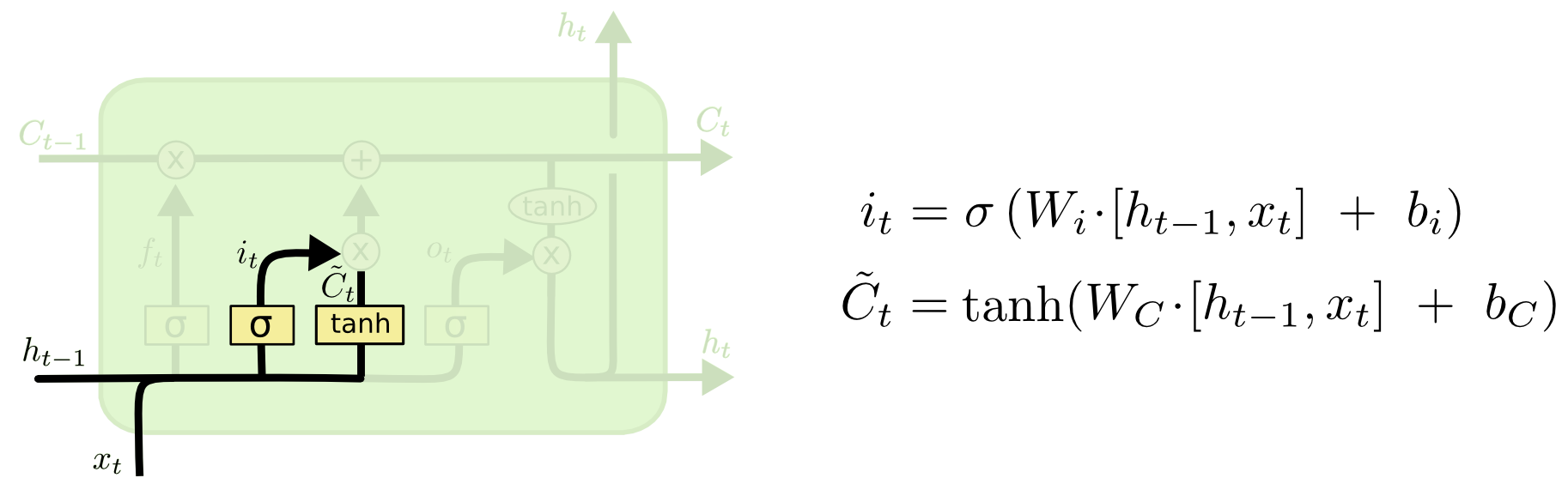The next step is to decide what new information we’re going to store in the cell state. This has two parts. First, a sigmoid layer called the "input gate layer" decides which values we’ll update. Next, a `tanh` layer creates a vector of new candidate values, C̃t, that could be added to the state. In the next step, we’ll combine these two to create an update to the state.

Not much to add here, we do more or less the same in both function, the only difference being the activation function (and the weights being used of course).

``````W_i, b_i = default_weights_and_bias()

# Input Gate Layer
#
# Shapes:
#   - W_i: 24x27
#   - ht_minus_1_and_xt: 27x?
#   - b_i: 24x1
#   - i_t: 24x?
def i_t(ht_minus_1_and_xt):
return tf.sigmoid(tf.matmul(W_i, ht_minus_1_and_xt) + b_i)

W_C, b_c = default_weights_and_bias()

# New Candidates for the Conveyor
#
# Shapes:
#   - W_C: 24x27
#   - ht_minus_1_and_xt: 27x?
#   - b_c: 24x1
#   - candidate_C_t: 24x?
def candidate_C_t(ht_minus_1_and_xt):
return tf.tanh(tf.matmul(W_C, ht_minus_1_and_xt) + b_c)
``````

### 3.3 Update Conveyor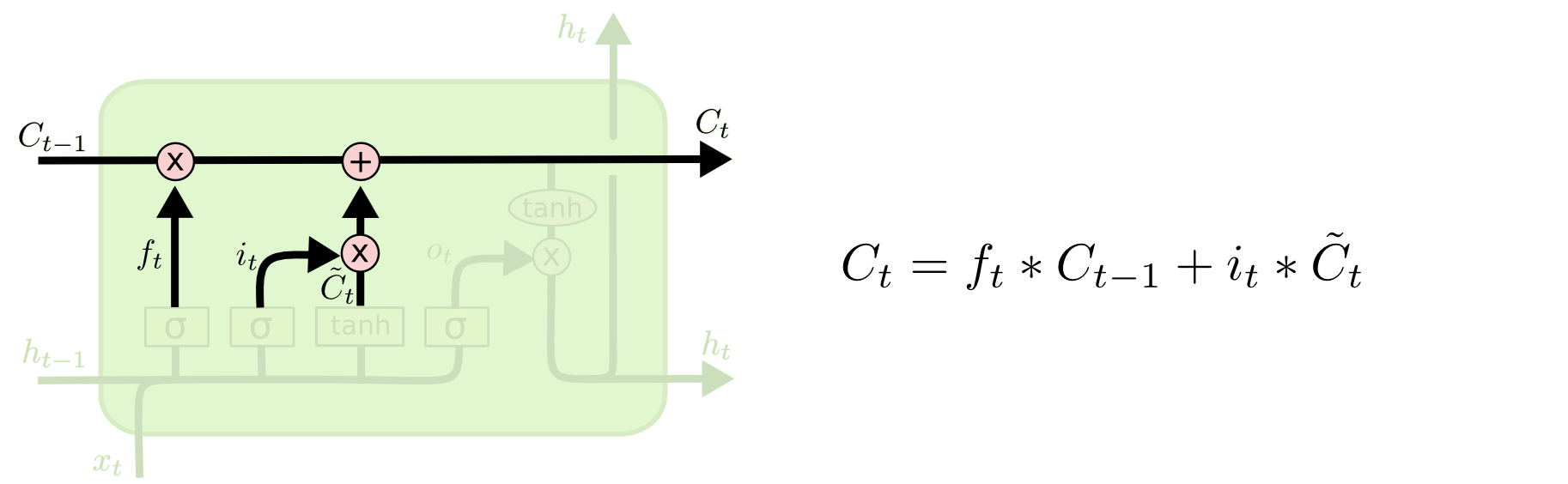It’s now time to update the old cell state, Ct−1, into the new cell state Ct. The previous steps already decided what to do, we just need to actually do it.

We multiply the old state by ft, forgetting the things we decided to forget earlier. Then we add it ∗ C̃. This is the new candidate values, scaled by how much we decided to update each state value.

``````# Updated Conveyor
#
# Shapes:
#   - f_t: 24x?
#   - Conveyor: 24x?
#   - i_t: 24x?
#   - CandidateConveyor: 24x?
def C_t(ht_minus_1_and_xt, Conveyor, CandidateConveyor):
return f_t(ht_minus_1_and_xt) * Conveyor + i_t(ht_minus_1_and_xt) * CandidateConveyor
``````

### 3.4 Prediction (for current LSTM step)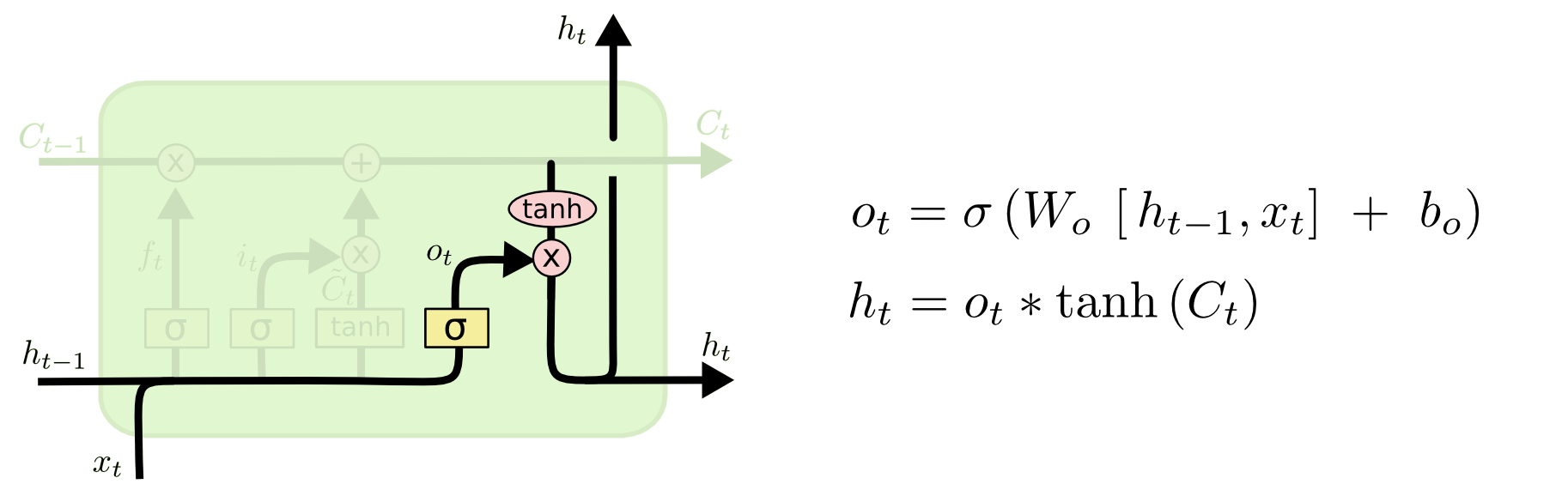Finally, we need to decide what we’re going to output. This output will be based on our cell state, but will be a filtered version. First, we run a sigmoid layer which decides what parts of the cell state we’re going to output. Then, we put the cell state through tanh (to push the values to be between −1 and 1) and multiply it by the output of the sigmoid gate, so that we only output the parts we decided to.

``````W_o, b_o = default_weights_and_bias()

# Updated Conveyor
#
# Shapes:
#   - W_o: 24x27
#   - b_o: 24x1
#   - ht_minus_1_and_xt: 27x?
#   - FinalConveyor: 24x?
#   - o_t: 24x?
#   - h_t: 24x?
def h_t(ht_minus_1_and_xt, FinalConveyor):
o_t = tf.sigmoid(tf.matmul(W_o, ht_minus_1_and_xt) + b_o)

return o_t * tf.tanh(FinalConveyor)
``````

### 3.5 The LSTM Cell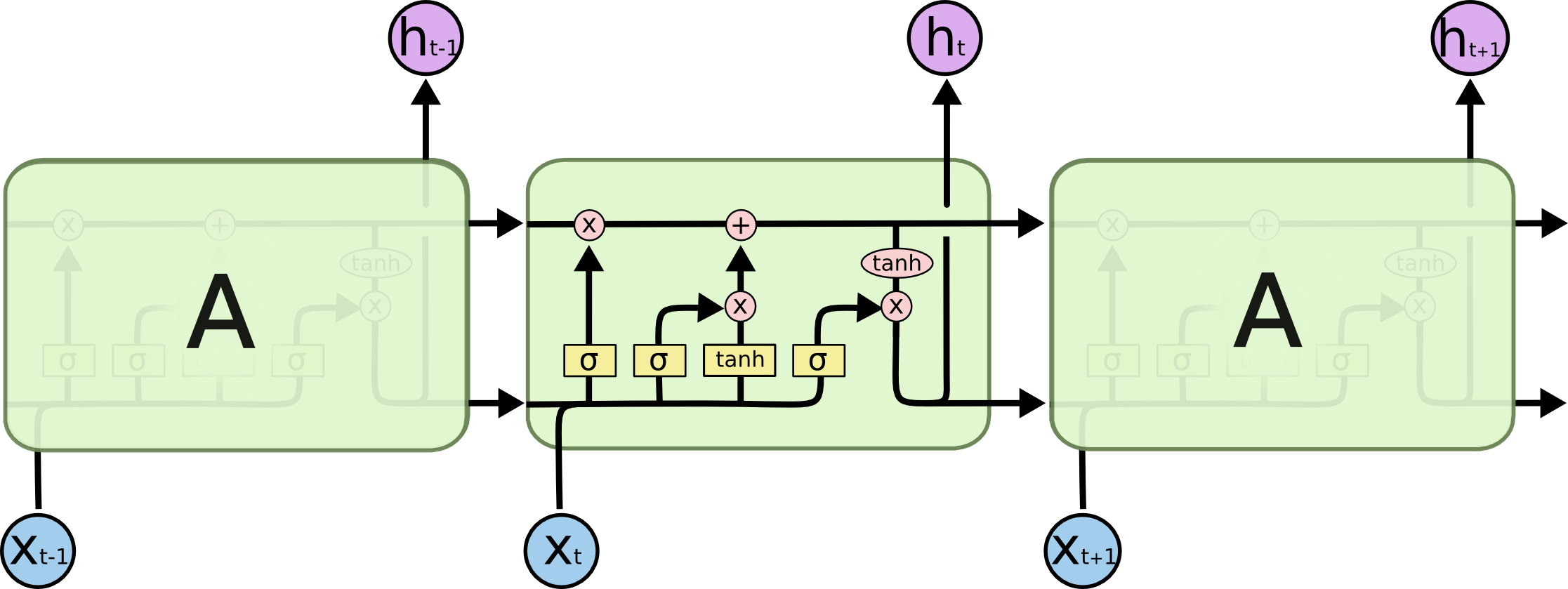Last but not least everything gets together in this method. Of course I could have saved me the trouble of putting every layer into a single function, but then I couldn't have split it up into multiple explainable steps.

One thing that's important here though is the tranpose operation of the input data - we need to do this in order to get the multiplication operations above working.

``````def lstm_cell(ht_minus_1_and_Conveyor, xt):
ht_minus_1, Conveyor = ht_minus_1_and_Conveyor

ht_minus_1_and_xt = tf.transpose(tf.concat([ht_minus_1, xt], 1))

CandidateConveyor = candidate_C_t(ht_minus_1_and_xt)

FinalConveyor = C_t(ht_minus_1_and_xt, Conveyor, CandidateConveyor)

lstm_prediction = tf.transpose(h_t(ht_minus_1_and_xt, FinalConveyor))

return(lstm_prediction, FinalConveyor)
``````

Finally we need to loop through every "timestep". The idea of our sequence-sum game is that we want to be able to play arbitrarily long sequences, so we need to go through step by step. TensorFlow's `while_loop` is a bit quirky, but it works.

``````data_length = tf.shape(data)

# This loop gets called once for every "timestep" and obtains one column of the input data
def lstm_loop(last_lstm_prediction, last_state, step):
lstm_prediction, state = lstm_cell((last_lstm_prediction, last_state), data[:, step, :])

initial_Conveyor = tf.zeros([LSTM_SIZE, data_length])
initial_prediction = tf.zeros([data_length, LSTM_SIZE])

timesteps = sequence_length

for_each_time_step = lambda a, b, step: tf.less(step, timesteps)

lstm_prediction, lstm_state, _ = tf.while_loop(for_each_time_step, lstm_loop, (initial_prediction, initial_Conveyor, 0), parallel_iterations=32)
``````

When we're through with the LSTM-Part we're left with 24 nodes. We collapse them to one final output node with a last set of weights and biases and get our prediction.

``````weight = tf.Variable(tf.truncated_normal([LSTM_SIZE, 1]))
bias = tf.Variable(tf.constant(0.1, shape=))

prediction = tf.matmul(lstm_prediction, weight) + bias
``````

## 4. Cost & Optimizing

Nothing special here, just standard optimization stuff.

``````with tf.name_scope('mean_square_error'):
mean_square_error = tf.reduce_sum(tf.square(tf.subtract(target, tf.unstack(prediction, axis = 1))))
tf.summary.scalar('mean_square_error', mean_square_error)
``````
``````<tf.Tensor 'mean_square_error_1:0' shape=() dtype=string>
``````
``````optimizer = tf.train.AdamOptimizer()
minimize = optimizer.minimize(mean_square_error)
``````
``````with tf.name_scope('error'):
with tf.name_scope('mistakes'):
mistakes = tf.not_equal(target, tf.round(tf.unstack(prediction, axis = 1)))
with tf.name_scope('error'):
error = tf.reduce_mean(tf.cast(mistakes, tf.float32))
tf.summary.scalar('error', error)
``````
``````<tf.Tensor 'error_1:0' shape=() dtype=string>
``````

## 5. Training

This should also be pretty familiar to you. You can run `tensorboard --logdir=logs` to get some nice graphs.

``````sess = tf.InteractiveSession()
merged = tf.summary.merge_all()

date = str(datetime.datetime.now())
train_writer = tf.summary.FileWriter('logs/selfmade_lstm/' + date + '/train', sess.graph)
test_writer = tf.summary.FileWriter('logs/selfmade_lstm/' + date + '/test', sess.graph)

init_op = tf.global_variables_initializer()
sess.run(init_op)
``````
``````epoch = 4000

for i in range(epoch):
if (i + 1) % 20 == 0:
summary, incorrect, mean_squ_err = sess.run([merged, error, mean_square_error], {data: test_input, target: test_output})

print('Epoch {:4d} | incorrect {: 3.1f}% | mean squ error {: 3.1f}'.format(i + 1, incorrect * 100, mean_squ_err))
else:
summary, acc = sess.run([merged, error], {data: train_input, target: train_output})

sess.run(minimize,{data: train_input, target: train_output})
``````
``````Epoch   20 | incorrect  77.4% | mean squ error  8864.7
Epoch   40 | incorrect  77.2% | mean squ error  8195.1
Epoch   60 | incorrect  73.9% | mean squ error  7601.0
...
``````
``````
And that's it! You can now test the results with code like this – just replace the string with any combination of `+`, `-`, `0` and `I`. The string has to be 6 characters though, but it shouldn't be too hard to edit our implementation so it takes an arbitrary amount of numbers – I leave that as an exercise to you though. Let me know if you did got it working :-)

```python
# Test the result
sess.run(prediction, {data: [data_generator.encodeSequence("00-+++")]})
``````
``````array([[ 1.98095763]], dtype=float32)
``````
``````sess.close()
train_writer.close()
test_writer.close()

``````